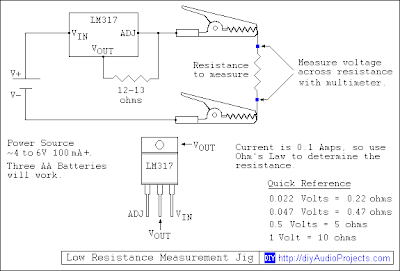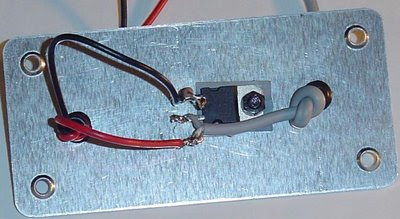## 26 November 2008

### Simple Low Resistance Measurement

This is a question that we get often:
I measured the direct current resistance (DCR) of xyz using a multimeter and the result is higher than what the data sheet says it should be!
Since most of our readers are audio enthusiasts, xyz is typically a speaker, but it could be any low resistance device such as a resistor, switch or relay contacts. When trying to make a resistance measurement of low resistance devices using a common multimeter, the results are often high and almost meaningless. The reason for this is that the test currents generated by most common multimeters are very low, typically much less than 1 milliamperes (mA).

A simple way to measure low resistance is to use a "4-wire (Kelvin) resistance measurement method". Ohm's Law states that V=IR. Resistance (R) is what we are interested in determining. Since we already have multimeter that is pretty accurate at measuring voltage (V), all we need to do is fix the current (I) and we can calculate the unknown resistance.

Figure 1 (click to enlarge) shows a schematic for a very simple low resistance measurement jig. Basically it is a LM317 voltage regulator that is set to deliver 100 mA of test current.Figure 1 - Low Resistance Measurement Circuit
In the circuit, a fixed 12 ohm resistor is used to set the current of the regulator to about 100 mA (a 12 ohm resistor actually sets it at 104 mA, use the LM317 current regulator calculator to check).

Building the Low Resistance Measurement Jig

The test jig is very simple to build. The photos below show the measurement jig that I built. The LM317 was recycled, a 12 ohm resistor, a couple of alligator clips, a project box (Radio Shack 270-1802) and an old 5V 300 mA cell phone charger. For the power source something in the order of 4 to 6 V capable of more than 100 mA will work fine. Batteries can also be used - as little as
three AA batteries will work. Note that a 9V battery will not work as it cannot deliver enough current.Photo 2 – LM317 and Set Resistor
Once you have built the jig, it is a good idea to check the test current to make sure it works. Mine produces a constant current of 105 mA. Anything between 95 and 105 mA will be fine as we will see later.

Using the Low Resistance Measurement Jig
Using the jig is very simple. Set your multimeter to read voltage. The lower you can set the voltage scale, the more accurate your readings will be. To determine the unknown resistance, simply connect the constant current across the device you want to measure. Now measure the voltage across the device you want to measure. Once you have measured a voltage you can apply Ohm's Law to determine the unknown resistance.

A 100 mA current was chosen for a reason - to make the math easy. With a 100 mA test current you can multiply the measured voltage by 10 to determine the unknown resistance. If you need very accurate low resistance measurements you can use the exact test current of your jig and an Ohm's Law Calculator to determine resistance.

Here are a few examples using the DIY low resistance test jig and a Wavetek Meterman multimeter:

0.1 ohm resistor measured 0.0112V (11.2mV), multiply by 10 and you get 0.112 ohms. In contrast, using the multimeter set to the lowest scale (200 ohm) 0.4 ohms was measured.

0.22 ohm resistor measured 0.0217V (21.7mV), multiply by 10 and you get 0.217 ohms. In contrast, using the multimeter set to the lowest scale (200 ohm) 0.5 ohms was measured.

0.47 ohm resistor measured 0.0481V (48.1mV), multiply by 10 and you get 0.481 ohms. In contrast, using the multimeter set to the lowest scale (200 ohm) 0.8 ohms was measured.

As you can see, for small resistance a common multimeter is very inaccurate. Of course you can spend a lot of money on a meter that can measure low resistance, but where is the fun in that? One should be able to put this jig together for less than \$10.

4-Wire (Kelvin) Resistance Measurement - Video Tutorial

More DIY Audio Prototyping Tools

1.When measuring a resistance with a digital multimeter, one of the most common mistakes made is not factoring in the resistance of the meter's leads. This will produce a false higher reading. By first clipping the two leads together you can measure the resistance of the meter, for example my multimeter lead resistance is 0.4 ohms. Then when you measure the actual resistance of interest, remember to subtract the lead's resistance to get the correct result.

2.@Martin

Yes and no. There is no way that 1 foot of test leads have a resistance of .4 ohms, not even if they were the size of a hair. The reason is that the test current produced by a meter is to small to measure low resistance accurately. Subtracting that amount helps, but it is still not accurate.

3.Yes, as noted, that will give you an improved reading, but still not accurate especially when you are trying to measure very low resistance.

4.Larry Holmes aka "kosimov"4:20 PM, August 09, 2010

And, as I have experienced many times, even when you null out the meter leads resistance, you still have a tough job ahead. Reason is: the wires are stranded. This means there are numerous smaller diameter solid wires connected in parallel, inside the insulation of the lead. These wires touch each other many times throughout the space inside the insulation, with the result that they have the effect of numerous very low value resistances connected in series and parallel, between the test probes and the meter plugs.

Why is that a problem? As you pick up the test probes and apply them to the resistance you wish to measure, all those series-parallel combinations change many times with each minute movement of the leads! That means the net resistance of the lead wires will also change, making the measurements you took to cancel out the lead's resistance a waste of time; as soon as you move the leads, the resistance changes.

The only good way to deal with this is to use some kind of four-wire method, where a constant current source supplies current to the resistance being tested though one set of stable wires, while the voltage across the resistance is measured with a totally separate pair of wires, thus, the voltage reading will not be affected by the very small resistance of the voltmeter's leads, because the drive current does not flow through them. There are many examples of this available on the internet; they show clever ways to do this without a great deal of trouble or expense.

5.Kelvin clips are easy to cobble. Cut off the metal pivot ears from standards clips and replace them with a small rod of plastic, thus insulating the two clip halves. Pull the jaws closed with a short length of rubber tube. Then wire one jaw to the current source and the other to the DVM. Presto! Now you are measuring low resistances accurately!

6.The Kelvin resistance method assumes the constant current source is really constant throughout the measurement. The LM317 is rated at 1.5 amps but trying to push 1 A with unspecified power source without proper heat sink will not get the actual current. Better use the old fashion method of having a current meter (ammeter) in the circuit, so you will know the current at all time. You will need two DMM, but DMM are so cheap now days.

7.Anonymous 6:32 AM, January 16, 2014:
"The LM317 is rated at 1.5 amps but trying to push 1 A"

Actually the LM317 voltage regulator is set to deliver 100 mA of test current, not 1A.

8.The many thousand dollar Hickock the USAF gave me to work on RADAR in the university of South East Asia, Class of '74 was a four wire unit. Two 8" 90* analog dials so I could match "G-lines" to .0001 ohm in F4's. They couldn't find "home" without it and AAA was homed in on RADAR signals.

9.Thank your tips it very easy to understand and I have used successfully.

10.Thanks for the info.

11.I was searching depth information about low resistance measurement. Because, I need this for my assignment. Really, you explain here quite well.

12.if i use 3 AA then what watt rating should the 12 ohm resistor be?

13.thank you for this great article , your post is a source of inspiration for me

14.At point m the meaured resistance it is higher than the actual value at and at point n it is lower than the actual value 7:20

15.Thank you.. I have apply it , and it worked successfully

16.17.18.